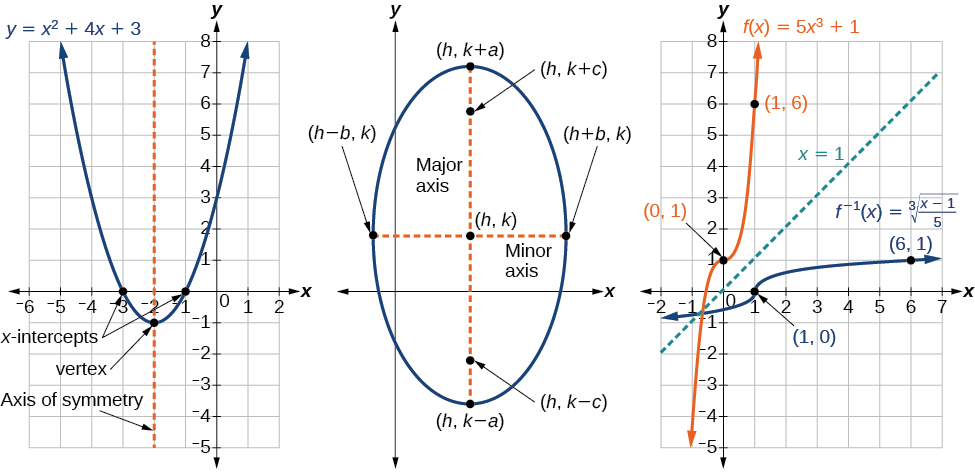# Preface  (Page 4/5)

 Page 4 / 5

## Example

Each learning objective is supported by one or more worked examples, which demonstrate the problem-solving approaches that students must master. Typically, we include multiple Examples for each learning objective in order to model different approaches to the same type of problem, or to introduce similar problems of increasing complexity. All told, there are more than 650 Examples, or an average of about 55 per chapter.

All Examples follow a simple two- or three-part format. First, we pose a problem or question. Next, we demonstrate the Solution, spelling out the steps along the way. Finally (for select Examples), we conclude with an Analysis reflecting on the broader implications of the Solution just shown.

## Figures

Openstax Precalculus contains more than 2000 figures and illustrations, the vast majority of which are graphs and diagrams. Art throughout the text adheres to a clear, understated style, drawing the eye to the most important information in each figure while minimizing visual distractions. Color contrast is employed with discretion to distinguish between the different functions or features of a graph.## Supporting features

Four small but important features, each marked by a distinctive icon, serve to support Examples.A “How To” is a list of steps necessary to solve a certain type of problem. A How To typically precedes an Example that proceeds to demonstrate the steps in action.A “Try It” exercise immediately follows an Example or a set of related Examples, providing the student with an immediate opportunity to solve a similar problem. In the Online version of the text, students can click an Answer link directly below the question to check their understanding. In other versions, answers to the Try-It exercises are located in the Answer Key.A Q&A may appear at any point in the narrative, but most often follows an Example. This feature pre-empts misconceptions by posing a commonly asked yes/no question, followed by a detailed answer and explanation.The “Media” icon appears at the conclusion of each section, just prior to the Section Exercises. This icon marks a list of links to online video tutorials that reinforce the concepts and skills introduced in the section.

Disclaimer: While we have selected tutorials that closely align to our learning objectives, we did not produce these tutorials, nor were they specifically produced or tailored to accompany Openstax Precalculus . We are deeply grateful to James Sousa for compiling his incredibly robust and excellent library of video tutorials, which he has made available to the public under a CC-BY-SA license at http://mathispower4u.yolasite.com/. Most or all of the videos to which we link in our “Media” feature (plus many more) are found in the Algebra 2 and Trigonometry video libraries at the above site.

## Section exercises

Each section of every chapter concludes with a well-rounded set of exercises that can be assigned as homework or used selectively for guided practice. With over 5900 exercises across the 12 chapters, instructors should have plenty to choose from 5,924 total exercises. Includes Chapter Reviews and Practice Tests. .

a colony of bacteria is growing exponentially doubling in size every 100 minutes. how much minutes will it take for the colony of bacteria to triple in size
what is the importance knowing the graph of circular functions?
can get some help basic precalculus
What do you need help with?
Andrew
how to convert general to standard form with not perfect trinomial
can get some help inverse function
ismail
Rectangle coordinate
how to find for x
it depends on the equation
Robert
whats a domain
The domain of a function is the set of all input on which the function is defined. For example all real numbers are the Domain of any Polynomial function.
Spiro
foci (–7,–17) and (–7,17), the absolute value of the differenceof the distances of any point from the foci is 24.
difference between calculus and pre calculus?
give me an example of a problem so that I can practice answering
x³+y³+z³=42
Robert
dont forget the cube in each variable ;)
Robert
of she solves that, well ... then she has a lot of computational force under her command ....
Walter
what is a function?
I want to learn about the law of exponent
explain this
what is functions?
A mathematical relation such that every input has only one out.
Spiro
yes..it is a relationo of orders pairs of sets one or more input that leads to a exactly one output.
Mubita
Is a rule that assigns to each element X in a set A exactly one element, called F(x), in a set B.
RichieRich
If the plane intersects the cone (either above or below) horizontally, what figure will be created?

#### Get Jobilize Job Search Mobile App in your pocket Now!ByByBy OpenStaxBy OpenStaxBy Sean WiffleBoyBy Stephen VoronBy OpenStaxBy JavaChamp TeamBy Stephanie RedfernBy OpenStaxBy OpenStaxBy OpenStax